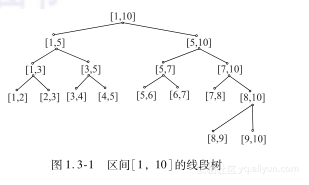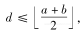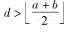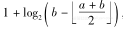#《程序设计解题策略》——1.3　利用线段树解决区间计算问题

0
0
01. 云栖社区>
2. 博客>
3. 正文

## 《程序设计解题策略》——1.3　利用线段树解决区间计算问题

### 1.3　利用线段树解决区间计算问题

1.3.1　线段树的基本概念【定理1.3-1】　线段树把区间上的任意一条线段都分成不超过2*log2L条线段。

1) 在区间(a，b)中，如果线段(c，d)满足(c≤a)或(d≥b)，则该线段在区间(a，b)中被拆分的线段数不超过log2(b-a)条。

① 若，则该线段拆分为它左儿子区间的线段，线段数不超过log2a+b2」-a，即不超过log2(b-a)，成立；
② 若，则相当于该线段被拆分为它左儿子区间的线段和右儿子区间的线段，线段数不超过，也不超过log2(b-a)，成立。

2) 在区间(a，b)中，对于任意线段也用归纳法证明。

struct Node　　　　　　　　　　　　　　　　　　// 节点的结构定义
{
int a,b,left,right,cover;// 代表区间［a,b］；指向左右子树的指针分别为left和
// right；节点基数（即覆盖［a,b］的线段数）为cover
} Tree［Maxn］;// 数组Tree［］存储线段树，数组元素的类型定义为Node，
// 数组下标为节点序号

1.3.2　线段树的基本操作和拓展

1) 建立线段树T(a，b)。
2) 将区间［c，d］插入线段树T(a，b)。
3) 将区间［c，d］从线段树T(a，b)中删除。
4) 线段树的动态维护。

（1） 建立线段树T(a，b)

void build(int a,int b)
{
int Now;
Tot++;Now=Tot;// 代表区间［a，b］的变量初始化
Tree［Now］.a=a;Tree［Now］.b=b;Tree［Now］.cover=0;
if(b-a>1)// 若该区间存在
{
int mid=(a+b)>>1;// 计算中间指针
Tree［Now］.left=Tot+1;build(a,mid);// 给左儿子编号并递归左子区间
Tree［Now］.right=Tot+1;build(mid,b);// 给右儿子编号，并递归右子区间
}
}


（2） 将区间［c，d］插入以Num节点为根的线段树

void insert(int Num)// 将区间［c,d］插入以Num为根的线段树
{
if(c<=Tree［Num］.a&&Tree［Num］.b<=d)// 若区间［c,d］覆盖v节点代表的区间，则覆盖该区间的
// 线段条数+1
Tree［Num］.cover++;
Else// 否则，若［c,d］不跨越区间中点，就只对左树或右树上进行
// 插入；若［c,d］跨越区间中点，则在左树和右树上都要进行插入
{
int mid=(Tree［Num］.a+Tree［Num］.b)>>1;
if(c<=mid) insert(Tree［Num］.left);
if(d>=mid) insert(Tree［Num］.right);
}
}


（3） 将区间［c，d］从以Num节点为根的线段树中删除

void del(int Num)// 从以Num节点为根的线段树中删除区间［c,d］
{
if(c<=Tree［Num］.a&&Tree［Num］.b<=d)// 若区间［c,d］覆盖Num节点代表的区间，则覆盖该区间的
// 线段条数-1；在区间［c,d］不覆盖Num节点代表的区间的情况下，若［c,d］不跨越区间中点，就只对左树或右树
// 上进行删除；否则在左树和右树上都要进行删除
Tree［Num］.cover--;
else
{
int mid=(Tree［Num］.a+Tree［Num］.b)>>1;
if(c<=mid)del(Tree［Num］.left);
if(d>=mid)del(Tree［Num］.right);
}
}


（4） 线段树的动态维护

void Count(int Num)
{
if(Tree［Num］.cover)Number+=(Tree［Num］.b-Tree［Num］.a);
else
{
if(Tree［Num］.left)Count(Tree［Num］.left);
if(Tree［Num］.right)Count(Tree［Num］.right);
}
}

类似的，还有求并区间的个数等。这里不再深入列举。
（5） 线段树的合并

<div style="text-align: center">
<img src="https://yqfile.alicdn.com/f4f187c041c3c091ee1e7e81018e9faa3165a9c6.png " >
</div>

void Merge（a,b）:
{ 如果a、b中有一个不含任何元素，就返回另一个；

}

由于a和b两棵线段树的树结构相同，上面过程的正确性是显然的。a、b中可能存在key相同的元素，之前对线段树的定义于这种情况无能为力，所以需要一个merge_leaf过程给出新树中该位置的元素。为了方便确定一棵树是否为空，动态开辟节点。若某棵子树为空，则其对应的父亲指针为空。例如，图1.3-3给出了合并两棵线段树后维护区间内数字个数的样例。
<div style="text-align: center">
<img src="https://yqfile.alicdn.com/2cdf35f87ab288370a842c8ff2157ace253839ed.png " >
</div>

1) merge_leaf过程。
2) 连接操作。
3) merge过程。
4) make_leaf过程。

**1.3.3　应用线段树解题**

1) 分析试题，揭示问题的区间特征。
2) 根据要求构建线段树。一般情况下需要对坐标离散。建树时，不要拘泥于问题的原型是线段还是数值或是其他什么形式，只要能够表示成区间，而且区间是由单位元素(可以是一个点、一条线段或数组中一个值)组成的，都可以采用线段树模型；不要局限于一维，可以根据题目要求建立二维的面积树、三维的体积树等。关于二维面积树的计算，我们将在4.1.2节中介绍。
3) 线段树的每个节点可以根据题目所需，设置记录区间线段特征值的变量。
4) 用树型结构来维护这些变量：如果是求节点总数，则是左右子树的节点数和+1(加上子根)；如果要求最值，则是左右子树的最值再联系本区间。利用每次插入、删除时仅修改O(log2L)个节点这个特点，在O(log2L)的时间内维护修改后相关节点的变量。
5) 在非规则的删除操作和大规模修改数据的操作中，可以利用叶节点反映出区间内各种数据单一性的本质特征，灵活地将子树收缩为叶子或让被收缩的叶子释放还原出区间，从而避免重复操作，提高算法效率。

【1.3.1　Snake】
【问题描述】

1) 这条折线必须闭合。
2) 折线每段的端点只能是这给出的N个点。
3) 折线交于一点的两条线段构成90度角。
4) 折线的线段要与坐标轴平行。
5) 所有线段除在这N个点外，都不会相交。
6) 所有线段的长度之和必须最短。

<div style="text-align: center">
" >
</div>

<div style="text-align: center">
<img src=" https://yqfile.alicdn.com/271eb22d1538ee7a2695305629f6a59afeb23df8.png" >
</div>

<div style="text-align: center">
</div>

<div style="text-align: center">
<img src=" https://yqfile.alicdn.com/f12ca96696f7c7d0e336f4095bb38fbc6d4f587a.png" >
</div>

<div style="text-align: center">
<img src="https://yqfile.alicdn.com/d036d5c6854b00fdc4f7b5ab882304efb67e7f93.png " >
</div>

# include

using namespace std;

const int MAXN = 100000;　　　　　　　　　　　// 点数的上限

struct ptype{// 点序列的元素类型
int x, y, id;// 点坐标为（x,y），输入顺序为id
};

struct stype{// 线段表的元素类型
ptype p1, p2;// 线段两个端点的坐标
};

ptype p［MAXN］;// 点序列
stype s［MAXN*2］;// 线段表，其中第i条线段为
int pos［MAXN］［4］;// 连接点i的线段条数为pos［i,0］，其中第j条线段的
// 序号为pos［i,j］
int a［2 MAXN］, aa［2 MAXN］;// y坐标序列
int tree［3 * MAXN］;// y轴区间的线段树
int e［MAXN］［4］;// 连接点i的线段条数为e［i,0］，其中第j条线段的
// 另一个端点序号为e［i,j］
bool f［MAXN］;// 事件点的访问标志序列
int n, m, sum, k, t;// 点数为n，最小长度为sum

void Init()// 输入信息
{
cin >> n;// 读入点数
for (int i = 1; i <= n; ++i)// 读入每点坐标,记下输入顺序
{ cin>>p［i］.x>>p［i］.y;p［i］.id=i; }
}

bool Succ()// 构造线段，若线段间衔接，则返回最小长度和成功标志
{
int i, j, t;
memset(s,0,sizeof(s)); m=0;sum=0;// 线段序列、线段条数和最小长度初始化
// 点l..点n以y为第一关键字、x作为第二关键字的
// 顺序排列；
t = 1;// 当前水平线段的点数初始化为1
for (i = 2; i <= n; ++i)// 由左而右搜索点2..n
{
if (p［i］.y != p［i - 1］.y)// 在i点为一条水平线段起点的情况下，若点数为奇数，
// 则失败退出；否则当前水平线段的点数设为1
{
if (t % 2 != 0) return false;
t = 1;
}
Else// i点与i-1点同属一条水平线段，水平线段的点数+1。
// 若点数为偶数，则添加一条连接i点与i-1点的线段，累计线段总长度，记下线段的序号和两个端点
{
if ((++t) % 2 == 0)
{
sum += sum　［i］.x-p［i-1］.x;
s［++m］.p1 = p［i-1］; s［m］.p2=p［i］;
pos［p［i］.id］［++pos［p［i］.id］［0］］=m;
pos［p［i-1］.id］［++pos［p［i-1］.id］［0］］=m;
e［p［i］.id］［++e［p［i］.id］［0］］=p［i-1］.id;
e［p［i-1］.id］［++e［p［i-1］.id］［0］］=p［i］.id;
}
}
}
if (t % 2 != 0) return false;// 若当前水平线段的点数为奇数，则失败退出
// 点l..点n以x为第一关键字、y作为第二关键字的
// 顺序排列
t = 1;// 当前垂直线段的点数初始化为1
for (i = 2; i <= n; ++i)// 由上而下搜索点2..n
{
if (p［i］.x != p［i - 1］.x)// 在i点为一条垂直线段起点的情况下，若点数为奇数，
// 则失败退出；否则当前垂直线段的点数设为1
{
if (t % 2 != 0) return false;
t=1;
}
else// i点与i-1点属同一条垂直线段。当前垂直线段的点数
// +1。若点数为偶数，则添加一条连接i点与i-1点的垂直线段。累计线段总长度，记下线段的序号和两个端点
{
if ((++t) % 2 == 0)
{
sum += p［i］.y-p［i-1］.y;
s［++m］.p1=p［i-1］;s［m］.p2=p［i］;
pos［p［i］.id］［++pos［p［i］.id］［0］］ = m;
pos［p［i-1］.id］［++pos［p［i-1］.id］［0］］=m;
e［p［i］.id］［++e［p［i］.id］［0］］=p［i-1］.id;
e［p［i-1］.id］［++e［p［i-1］.id］［0］］=p［i］.id;
}
}
}
if(t%2!=0)return false;// 若当前垂直线段的点数为奇数，则失败退出否则成功
// 退出
return true;
}

void find(int i)// 从点1出发,递归计算连通的点数k
{
++k; f［i］ = true;// 累计访问的点数,访问点i
if (! f［e［i］［1］］) find(e［i］［1］);// 若连接点i的第1条线段的另一端点未访问,则访问该点；
// 否则若连接点i的第2条线段的另一端点未访问,则访问该点
else
{
if (!f［e［i］［2］］) find(e［i］［2］);
}
}

bool Check1()// 返回所有线段是否连通的标志
{
k=0;memset(f,0, sizeof(f));// 访问的点数和访问标志初始化
find(1);// 从点1出发递归计算连通的点数k
if (k != n)return false;// 若连通的点数不足n,则返回false；否则返回true
else return true;
}

int cal(int x)// 在a数组中二分查找值为x的元素下标
{
int i, j, mid;
i=1;j=t;// 左右指针初始化
while (i != j)// 若区间存在,则计算中间指针
{
mid = (i + j) / 2;
if (a［mid］ == x) { i=mid;break; };// 若中间元素值为x,则返回其下标
if (x}
return i;// 返回a数组中值为x的元素下标
}

void tree_ins(int i,int s,int t,int k,int x)// 线段树中事件点i对应的区间为［s,t］。将其子树中
// 事件点k对应的子区间增加x个点
{
int mid;
if (s==t) { tree［i］ += tree［i］;return; }// 若找到事件点k，则对应的子区间增加x个点，并返回
mid = (s+t) / 2;// 计算区间的中间指针，根据事件点k所在的子区间递归
// 左子树或右子树
if (k <= mid) tree_ins(2*i,s,mid,k,x);
else tree_ins(2*i+1,mid+1,t,k,x);
tree［i］ = tree［2i］ + tree［2i+1］;// 累计事件点i对应区间的点数
}

int tree_check(int i,int s,int t,int s1,int t1)// 线段树中事件点i对应的区间为［s,t］，查找其子树区间
// ［s1，t1］的点数
{
int mid;
if((s==s1)&&(t==t1))return tree［i］;// 若找到子树区间［s1，t1］，则返回区间内的点数
mid = (s+t) / 2;// 计算区间的中间指针，分三种情形（［s1，t1］在左子树、
// 在右子树、横跨左右子树）递归
if (t1<=mid) return tree_check(2*i,s,mid,s1,t1);
if (s1>mid) return tree_check(2*i+1,mid+1,t,s1,t1);
return tree_check(2i,s,mid,s1,mid)+tree_check(2i+1,mid+1,t,mid+1,t1);
}

bool Check2()// 若出现线段自交的情况，则返回false；否则返回true
{
int i, j;
int l, k1, k2;
for (i=1;i<=n;++i)aa［i］=p［i］.y;// 点l..点n按照y坐标值递增的顺序建立事件序列a
t=1; a［1］ = aa［1］;
for (i = 2; i <= n; ++i) if (aa［i］ != aa［i-1］) a［++t］=aa［i］;
memset(tree, 0, sizeof(tree));// 线段树为空
for (i = 1; i <= n; ++i)// 枚举每个点连接的所有边
{
for (j = 1; j <= pos［p［i］.id］［0］; ++j)
{
l = pos［p［i］.id］［j］;// 计算连接点i的第j条边的序号l
if(s［l］.p1.x==s［l］.p2.x)// 若边l为垂直线段，则计算两端y坐标值对应的事件点
// k1和k2
{
k1 = cal(s［l］.p1.y); k2 = cal(s［l］.p2.y);
if ((k1+1<=k2-1)%(tree_check(1,1,t,k1+1,k2-1)!=0))
// 在线段树中查找［k1+1，k2-1］。若区间内有点存在，
// 说明存在与线段l相交的线段，返回线段自交标志
return false;
}
else
{
k1 = cal(s［l］.p1.y);// 边l为水平线段。计算边l的p1端的y值对应a序列
// 的事件点k1。若点i为边l的左端点，则对应的事件点k1插入y轴区间的线段树中；若点i为边l的右端点，
// 则对应的事件点k1从y轴区间的线段树中删除
if (p［i］.x == s［l］.p1.x) tree_ins(1,1,t,k1,1) ;
else tree_ins(1,1,t,k1,-1);
}
}
}
return true;// 返回所有线段不自交标志
}

void Make()// 若线段满足要求，则输出最小长度；否则输出无解信息
{
if(! Succ()){ cout<< "-1"<< endl;return;}// 若存在线段不衔接情况，则失败退出
if(! Check1()){ cout<< "-1"<if(! Check2()){ cout<<"-1"<cout << sum << endl;// 输出最小长度和
}

int main()
{
Init();// 输入信息
Make();// 若线段满足要求，则输出最小长度；否则输出无解信息
return 0;
}

【1.3.2　LCIS】
【问题描述】

1) U A B：用B取代第A个数(下标从0开始计数)。
2) Q A B：输出在［A，B］中最长连续递增子序列(the Longest Consecutive Increasing Subsequence，LCIS)的长度。

<div style="text-align: center">
<img src=" https://yqfile.alicdn.com/027fce1d4a259f7d1a2fc674b203a9d3998ed309.png" >
</div>

1) 包含左端点的最长连续递增子长度lsum。
2) 包含右端点的最长连续递增子长度rsum。
3) 整个区间的最长递增子长度Msum。

1) 若左儿子最右边的值<右儿子最左边的值，则节点的3个域调整如下：

lsum=(左儿子的lsum==左儿子的len)？左儿子的len+右儿子的lsum：左儿子的lsum;
rsum=(右儿子的rsum==右儿子的len)？右儿子的len+左儿子的rsum：右儿子的rsum;
Msum=max{ 左儿子的rsum+右儿子的lsum，左儿子的Msum，右儿子的Msum，lsum，rsum);

2) 左儿子最右边的值≥右儿子最左边的值，则节点的3个域调整如下：

lsum=左儿子的lsum；rsum=右儿子的rsum；Msum=max{左儿子的Msum，右儿子的Msum};

查找区间［l，r］中最长递增子序列的长度也需十分小心。查找过程从根出发，自上而下查找。

# define rson pos<<1|1// 节点pos的右儿子序号

using namespace std;
constint MSUMN=100005;
struct node// 线段树节点的结构定义
{
int l,r;// 代表区间［l,r］
int msum;// 整个区间的最长递增子序列长度为msum，其中左端最长连续
// 递增子长度为lsum、右端最长连续递增子序列长度为rsum
int lsum,rsum;
int mid()// 计算区间［l,r］的中间指针
{
return (l+r)>>1;
}
};
node tree［MSUMN*4］;// 线段树
int num［MSUMN］;// 序列
inline void pushup(int pos)// 上传左右儿子信息，调整pos节点的3个域
{
tree［pos］.msum=msum(tree［lson］.msum,tree［rson］.msum);
// 上传左右儿子信息
tree［pos］.lsum=tree［lson］.lsum;tree［pos］.rsum=tree［rson］.rsum;
if(num［tree［lson］.r］// 若左儿子右端数字小于右儿子左端数字，则合并左右儿子
{
tree［pos］.msum=msum(tree［pos］.msum,tree［lson］.rsum+tree［rson］.lsum);
if(tree［lson］.lsum==tree［lson］.r-tree［lson］.l+1)
// 左子区间满足递增要求
tree［pos］.lsum+=tree［rson］.lsum;
if(tree［rson］.rsum==tree［rson］.r-tree［rson］.l+1)
// 右子区间满足递增要求
tree［pos］.rsum+=tree［lson］.rsum;
}
}

void build(int l,int r,int pos)// 构建以pos节点为根、代表区间［l,r］的线段树
{
tree［pos］.l=l;tree［pos］.r=r;// 设定节点pos的左右指针
if(l==r)// 若节点pos为叶子,则左端最长连续递增子长度、右端的最长
// 连续递增子长度，以及整个区间的最长递增子长度为1，返回
{
tree［pos］.lsum=tree［pos］.rsum=tree［pos］.msum=1;
return ;
}
int mid=tree［pos］.mid();// 计算pos代表区间的中间指针
build(l,mid,lson);build(mid+1,r,rson);// 递归pos的左右子树
pushup(pos);// 上传左右儿子信息，调整pos的3个域
}

Void update(int a,int b,int pos)// 从节点pos出发，单点更新将num［a］的值改为b
{
if(tree［pos］.l==tree［pos］.r)// 找到位置，该值并返回
{ num［a］=b; return ;}
int mid=tree［pos］.mid();// 计算pos代表区间的中间指
if(a<=mid) update(a,b,lson);// 更新位置在左区间，则递归左儿子；否则递归右儿子
else update(a,b,rson);
pushup(pos);// 上传左右儿子信息，调整pos节点的3个域
}

int queryL(int l,int r,int pos)// 检查pos节点左端最长递增子序列的长度：若不超过待查区间
// ［l，r］的长度，则返回左端最长递增子序列的长度；否则返回［l，r］的长度
{
if(r-l+1>=tree［pos］.lsum)return tree［pos］.lsum;
else return r-l+1;
}

int queryR(int l,int r,int pos)// 检查pos节点右端最长递增子序列的长度：若不超过待查区间
// ［l，r］的长度，则返回右端最长递增子序列的长度；否则返回［l，r］的长度
{
if(r-l+1>=tree［pos］.rsum) return tree［pos］.rsum;
else return r-l+1;
}

int query(int l,int r,int pos)// 从节点pos出发，查找区间［l,r］中最长递增子序列的长度
{
if(l==tree［pos］.l&&r==tree［pos］.r)return tree［pos］.msum;
// 若pos节点恰为待查区间，则返回pos节点的最长递增子序列长度
int mid=tree［pos］.mid();// 计算pos节点的中间指针
if(r<=mid)return query(l,r,lson);// 若pos的左儿子含待查区间，则递归左儿子；若pos的右儿子
// 含待查区间，则递归右儿子
else if(l>mid)return query(l,r,rson);
else// 待查区间［l,r］横跨pos的左右儿子。LCIS［l,r］=max{左
// 儿子的LCIS［l,mid］，右儿子的LCIS［mid+1,r］,左儿子的LCIS［l,mid］+右儿子的LCIS［mid+1,r］,│左儿子右端数据
// 与右儿子左端数据满足递增要求}
{
int res=0;
if(num［tree［lson］.r］res=msum(res,queryR(l,mid,lson)+queryL(mid+1,r,rson));
res=msum(res,query(l,mid,lson));
res=msum(res,query(mid+1,r,rson));
return res;
}
}

int main()
{
int n,m,t;
scanf("%d",&t);// 输入测试用例数
while(t--)// 依次处理每个测试用例
{
scanf("%d%d",&n,&m);// 输入序列长度和命令数
int i;
for(i=1;i<=n;i++)// 输入序列
scanf("%d",&num［i］);
build(1,n,1);// 构建线段树
char word［2］;// 命令字
for(i=1;i<=m;i++)// 依次处理命令
{
int a,b;
scanf("%s%d%d",word,&a,&b);// 输入命令字和参数
if(word［0］=='U')update(a+1,b,1);// 若单点更新命令，则将num［a+1］的值改为b
else// 查询命令
{
int x=query(a+1,b+1,1);// 计算和输出num［a+1］..num［b+1］区间中最长连续递增
// 子序列的长度
printf("%d＼n",x);
}
}
}
return 0;
}

我们在本节给出的线段树是一维的，即仅介绍了利用线段树处理简单的几何线段或数据区间的一般方法。实际上线段树的拓展性很广：例如线段树既可以处理线段问题，也可以转化为对数据点的操作，因为数据点也可以被理解为特殊的区间；另外，虽然一维线段树便于线性统计，但亦可以拓展为二维线段树和多维线段树，用于平面统计和空间统计。有关这方面的知识，我们将在第4章中专门阐释。`

+ 关注